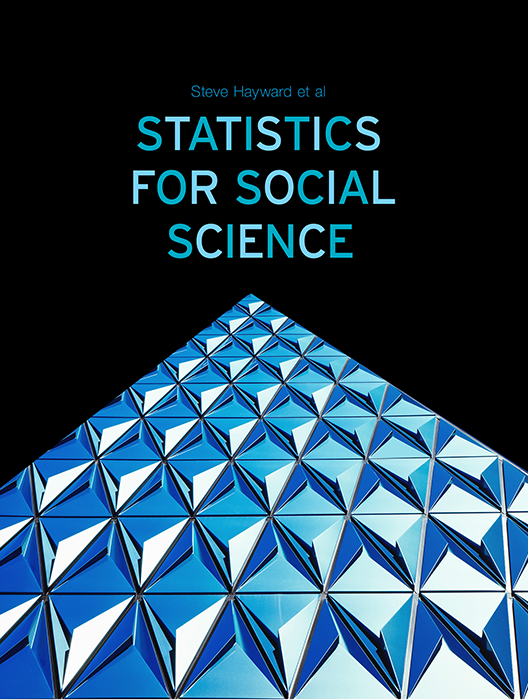Statistics for Social Science

Lead Author(s): Stephen Hayward

Statistics for Social Science takes a fresh approach to the introductory class. With learning check questions, embedded videos and interactive simulations, students engage in active learning as they read. An emphasis on real-world and academic applications help ground the concepts presented. Designed for students taking an introductory statistics course in psychology, sociology or any other social science discipline.

This content has been used by 8,525 students

What is a Top Hat Textbook?

Top Hat has reimagined the textbook – one that is designed to improve student readership through interactivity, is updated by a community of collaborating professors with the newest information, and accessed online from anywhere, at anytime.

• Top Hat Textbooks are built full of embedded videos, interactive timelines, charts, graphs, and video lessons from the authors themselves
• High-quality and affordable, at a significant fraction in cost vs traditional publisher textbooks

Key features in this textbook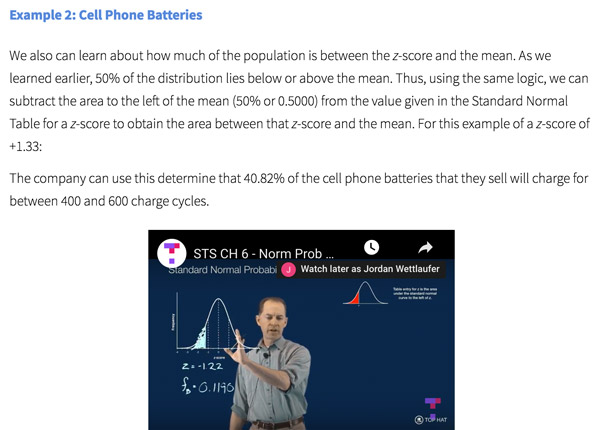Our Statistics for Social Science textbook allows students to manipulate data, visualize the effects discussed, and explore Lightboard videos that feature instructor explanations to reinforce concepts and calculations.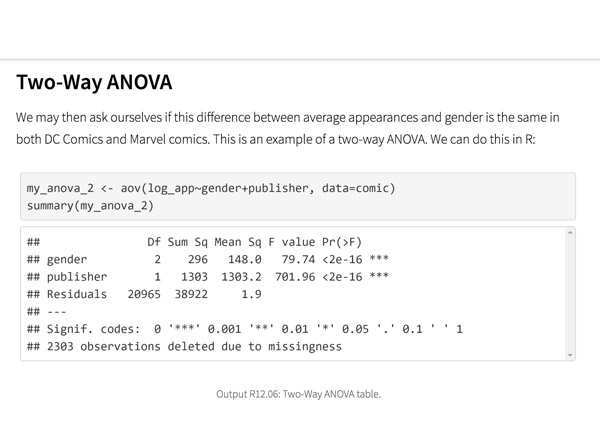Top Hat’s interactive offering includes a complementary module on using R software for data management, graphics, and conducting statistical analyses with examples and practice questions.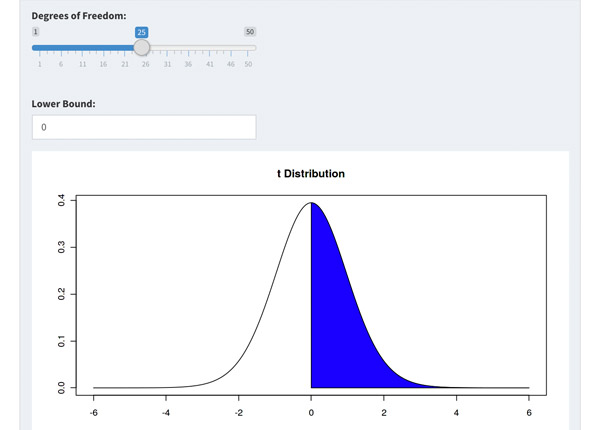Built-in assessment questions embedded throughout chapters so students can read a little, do a little, and test themselves to see what they know!

Comparison of Social Sciences Textbooks

Consider adding Top Hat’s Statistics for Social Sciences textbook to your upcoming course. We’ve put together a textbook comparison to make it easy for you in your upcoming evaluation.

Top Hat

Steve Hayward et al., Statistics for Social Sciences, Only one edition needed

Pearson

Agresti, Statistical Methods for the Social Sciences, 5th Edition

Cengage

Gravetter et al., Essentials of Statistics for The Behavioral Sciences, 9th Edition

Sage

Gregory Privitera, Essentials Statistics for the Behavioral Sciences, 2nd Edition

Pricing

Average price of textbook across most common format

Up to40-60%more affordable

Lifetime access on any device

$200.83 Hardcover print text only $239.95

Hardcover print text only

$92 Hardcover print text only Always up-to-date content, constantly revised by community of professors Content meets standard for Introduction to Anatomy & Physiology course, and is updated with the latest content In-Book Interactivity Includes embedded multi-media files and integrated software to enhance visual presentation of concepts directly in textbook Only available with supplementary resources at additional cost Only available with supplementary resources at additional cost Only available with supplementary resources at additional cost Customizable Ability to revise, adjust and adapt content to meet needs of course and instructor All-in-one Platform Access to additional questions, test banks, and slides available within one platform Pricing Average price of textbook across most common format Top Hat Steve Hayward et al., Statistics for Social Sciences, Only one edition needed Up to40-60%more affordable Lifetime access on any device Pearson Agresti, Statistical Methods for the Social Sciences, 5th Edition $200.83

Hardcover print text only

Pearson

Gravetter et al., Essentials of Statistics for The Behavioral Sciences, 9th Edition

$239.95 Hardcover print text only Sage McConnell, Brue, Flynn, Principles of Microeconomics, 7th Edition $92

Hardcover print text only

Always up-to-date content, constantly revised by community of professors

Constantly revised and updated by a community of professors with the latest content

Top Hat

Steve Hayward et al., Statistics for Social Sciences, Only one edition needed

Pearson

Agresti, Statistical Methods for the Social Sciences, 5th Edition

Pearson

Gravetter et al., Essentials of Statistics for The Behavioral Sciences, 9th Edition

Sage

Gregory Privitera, Essentials Statistics for the Behavioral Sciences, 2nd Edition

In-book Interactivity

Includes embedded multi-media files and integrated software to enhance visual presentation of concepts directly in textbook

Top Hat

Steve Hayward et al., Statistics for Social Sciences, Only one edition needed

Pearson

Agresti, Statistical Methods for the Social Sciences, 5th Edition

Pearson

Gravetter et al., Essentials of Statistics for The Behavioral Sciences, 9th Edition

Sage

Gregory Privitera, Essentials Statistics for the Behavioral Sciences, 2nd Edition

Customizable

Ability to revise, adjust and adapt content to meet needs of course and instructor

Top Hat

Steve Hayward et al., Statistics for Social Sciences, Only one edition needed

Pearson

Agresti, Statistical Methods for the Social Sciences, 5th Edition

Pearson

Gravetter et al., Essentials of Statistics for The Behavioral Sciences, 9th Edition

Sage

Gregory Privitera, Essentials Statistics for the Behavioral Sciences, 2nd Edition

All-in-one Platform

Access to additional questions, test banks, and slides available within one platform

Top Hat

Steve Hayward et al., Statistics for Social Sciences, Only one edition needed

Pearson

Agresti, Statistical Methods for the Social Sciences, 5th Edition

Pearson

Gravetter et al., Essentials of Statistics for The Behavioral Sciences, 9th Edition

Sage

Gregory Privitera, Essentials Statistics for the Behavioral Sciences, 2nd Edition

Steve HaywardRio Salado College

A lifelong learner, Steve focused on statistics and research methodology during his graduate training at the University of New Mexico. He later founded and served as CEO of Center for Performance Technology, providing instructional design and training development support to larger client organizations throughout the United States. Steve is presently lead faculty member for statistics at Rio Salado College in Tempe, Arizona.

Joseph F. Crivello, PhDUniversity of Connecticut

Joseph Crivello has taught Anatomy & Physiology for over 34 years, and is currently a Teaching Fellow and Premedical Advisor of the HMMI/Hemsley Summer Teaching Institute.

Explore this textbook

Read the fully unlocked textbook below, and if you’re interested in learning more, get in touch to see how you can use this textbook in your course today.

Single-Sample Hypothesis TestingBaseball is one of the most statistics heavy sports. Single-sample tests are often used to compare individual player's performances during a particular season to their career averages. ​

Chapter Objectives

After completing this chapter, you will be able to:

• Describe characteristics of a single-sample and determine when single-sample hypothesis testing is appropriate.
• Explain the steps involved in single-sample hypothesis testing.
• Choose the appropriate single-sample tests for large vs. small samples.
• Perform single-sample z-tests of means and proportions.
• Perform single-sample t-tests of means.
• Report and interpret results of single-sample hypothesis tests.

Introduction

In the last chapter, you learned about the logic of hypothesis testing. In this chapter, we will explore specific applications of hypothesis testing: namely, discovering how a single-sample mean compares to a population mean, both when the population standard deviation (σ) is known and when it is not known. We will learn single-sample hypothesis testing with large samples, using the z-score distribution, and with small samples, using the t-score distribution. We will also learn how to test the statistical significance of proportions. Don’t worry! There will be practice opportunities as well as demonstrations of practical applications of single-sample hypothesis testing. Take a deep breath and let’s begin!

The Logic of Single-Sample Hypothesis Testing

What is a single-sample? You will recall from earlier chapters that a sample is a subset of a larger population. Sometimes, researchers may want to know how a sample statistic compares to a known population parameter. For example, you may want to know if you and your friends consume more alcohol per week than typical American college students. Educators may want to know how the average reading performance of kindergarteners in a particular school compares to national reading scores. We can use single-sample hypothesis testing techniques to answer such questions about statistical inference.

Consider the graph below, which represents two sets of data. The blue curve has a standard score mean of zero, while the red curve has a mean slightly below that. Is that difference statistically significant? For example, if the blue curve represents a known population, is the sample represented by the red curve really (reliably) different from the population, or is the difference most likely just due to chance variation? How would you know? Here again, single-sample hypothesis testing can help us answer such a question.Figure 10.1: Comparison of a sample distribution to a known population distribution

In the scenarios below, indicate whether single-sample hypothesis testing would be appropriate by responding “yes” or “no.”

10.01

Comparison of high school males and females on amount of marijuana smoked during summer vacation.

A

Yes

B

No

10.02

A sociologist wants to determine how the survival rate for newborns in a small developing country compares to the rate reported by World Health Organization for heavily industrialized countries.

A

Yes

B

No

10.03

You wonder if the proportion of female to male psychology majors at your university is similar to that found in a national survey of university students.

A

Yes

B

No

10.04

A researcher wants to determine whether Hispanic and Native American men have similar startle responses when presented with an unexpected stimulus event.

A

Yes

B

No

Steps of Hypothesis Testing

The steps of hypothesis testing are summarized in the table below.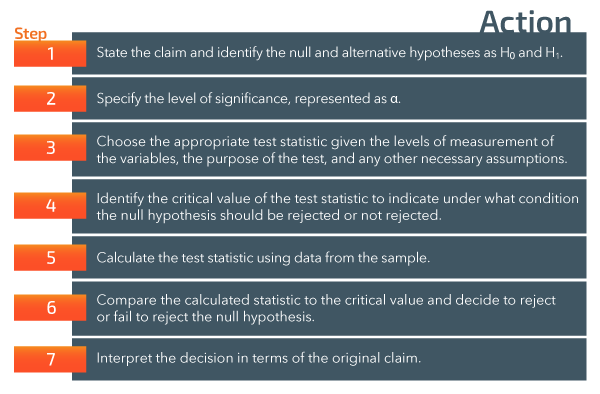Figure 10.2: Steps involved in hypothesis testing​

Here are some helpful tips as you think about how to go about conducting the hypothesis test:

• State the claim and identify the null (H0) and alternative (H1) hypotheses. Remember, H0 is the ‘no difference’ hypothesis or a statement of equality. Be sure to state the hypotheses so that they are complementary, as shown in the table below.

• Determine the alpha level (α). Is there a standard alpha level in the field? Consider the consequences of making a Type I or Type II error when determining the appropriate alpha level. Are you conducting a 1-tailed or a 2-tailed test?
• Determine the appropriate test to use. This will depend upon your answers to specific questions: What type of sample data do you have? A mean? A proportion? What do you know about the population parameters? The mean? Standard error of the mean? Proportion of participants who comprise a particular group? Answers to questions like these will guide your choice of statistical tests and computational formulas.
• Identify the critical value of the test statistic. How will that be calculated? What table or technology tool will you use?
• Calculate the test statistic. This step involves using the computational formula to calculate the appropriate test statistic or using a technology tool (either a statistical software package or a statistics calculator that can calculate and display the desired statistic).
• Compare the calculated test statistic to the critical value. If the calculated test statistic is more extreme than the critical value, reject H0. Otherwise, fail to reject H0.
• An alternative approach is to determine the chance probability of the value of the statistic, also known as the P-value. If the chance probability, or P-value, is > α, you must fail to reject H0. If the P-value is < α, you should reject H0.
• Interpret the decision. Summarize the finding in words. State a conclusion and make any inferences that are appropriate. Be as detailed as possible in your explanation.

10.05

Arrange the hypothesis testing steps in their correct order.

A

Decide to reject or fail to reject H$_0$

B

Interpret the decision in terms of the original claim

C

State the null hypothesis

D

Identify the critical values of the test statistic

E

Calculate the test statistic

F

Determine the appropriate statistical test

G

Determine the alpha level

Stating the Hypotheses

The null hypothesis states that the sample statistic (e.g. mean, standard deviation, proportion, etc.) does not differ from the hypothesized or known population parameter or differs in a way that is not predicted by the alternative hypothesis. Recall that the alternative hypothesis summarizes the outcome predicted or being tested by the research study.

A null hypothesis may be written as an equality between a particular statistic and a parameter. For example, x̅ = μ, x̄ ≥ μ, x̅ ≤ µ, or as an expected value for a statistic based on a population parameter,  e.g. x̅ = 250.

In order for the null to be complementary to the alternative hypothesis, equalities should usually be expressed as =, ≤, or ≥, since the alternative in these cases is usually ≠, >, or <. Stating them in complementary terms ensures that all possible outcomes of the study are taken into consideration.

Left-, Right-, or Two-Tailed?

The type of hypothesis test that is used is determined by the form of the alternative hypothesis. It corresponds to the directionality of the hypothesis statement, which in turn reflects the logic of the research being undertaken.Figure 10.4: Directionality of hypothesis statements​​

Summary of Logic

Left-tailed Test

If the researcher is conducting a significance test to see if the result that is obtained is reliably less than the population parameter, the test would be left-tailed because the outcome will be evaluated at the left tail of the sampling distribution. Only an outcome in the lower tail of the distribution of possible scores could be regarded as a “less than” result. In other words, only a computed statistic lower than the test statistic critical value results in the decision to reject H0.

Right-Tailed Test

In a similar vein, if the researcher is conducting a significance test to see if the result that is obtained is reliably greater than the population parameter, the test would be right-tailed because we would be evaluating the outcome at the right tail of the sampling distribution. Only an outcome in the upper tail of the distribution of possible scores could be regarded as a “more than” result. Only a computed statistic higher than the test statistic critical value results in the decision to reject H0.

Two-Tailed Test

If the alternative hypothesis does not predict a specific direction for the difference, an outcome in either tail of the distribution of possible scores could be regarded as a “not-equal-to” result leading to rejection of H0.

Z-Score Review

You will recall that z-scores are standard scores which indicate a raw score’s distance from the mean in standard deviation units. A raw score equal to the mean would have a z-score of 0. Whereas the z-score associated with a raw score 1 standard deviation above the mean would equal 1.0, the z-score associated with a raw score 1 standard deviation below the mean would equal -1.0. Recall also that the proportion of area under the normal curve between any two z-scores is equal to the probability of obtaining a score in that region by chance. The figure below depicts the standard normal curve with z-score equivalents on the x-axis, proportions of area under the curve between the dotted lines on the graph, and grouped areas under the curve summarized below the x-axis. For example, the proportion of area under the standard normal curve between the mean and a score that is one standard deviation above or below the mean is .3413. Reminder: z-scores can be positive or negative, but area and probability are always positive. You also learned that .025 or 2.5% of the area under the curve falls beyond a z-score equivalent of 1.96. A z-score of +/-1.96 corresponds to a two-tailed α level of .05 because the z values of +1.96 and -1.96 enclose 95% of the area under the curve, as you can see in the graphic example below.Figure 10.5: Proportion of area in the critical region of the standard normal curve for α= 0.05​​

We can see that only 5 times out of 100 would you expect to get a z-value of more than +1.96 or less than -1.96. Remember that the extreme 5% would actually be split between the two tails of the distribution—2.5% in one tail and 2.5% in the other tail. Let’s make sure we begin thinking of extreme scores or values as basically things that have a very low probability of occurring.

Testing a Hypothesis About the Mean

The standardized test statistic to be used for a hypothesis test of a mean depends on the amount of information that is available to the researcher. Here is a decision table to use:

Large Sample Testing Using the Z-test

If the population can be assumed to be normally distributed and the standard deviation σ is known, or if not known and n ≥ 30, the z-test can be used to test the null hypothesis for the mean of a single sample.

Population Standard Deviation is Known

The standard z-score formula for hypothesis testing of single sample means when the population is normally distributed and the standard deviation is known (any sample size) is written as follows:

where σ / √n is the formula for the standard error of the mean, represented as σ

Sample Size n ≥ 30

If the sample size n is ≥ 30, the sample is considered large and the standard deviation s can be used as an estimate of the population standard deviation σ. The standard z-score formula for hypothesis testing of single sample means when the sample size n is ≥ 30 is written as follows:

In the formulas above, x̅ represents the sample mean, μ is the known or hypothesized population mean, and s / √n estimates the standard error of the mean. A standard score (z) will reflect the distance in standard deviation units of a sample mean from a population mean. We can then use the z-score table and proportion of area under the standard normal curve to compute the likelihood that the sample mean was drawn from the population. In other words, the z-score statistic will tell us the distance our sample mean is from the population mean. We can then determine if the sample mean is statistically significantly different from the population mean, in which case we would reject H0.

Example

In a recent study, Lepp, Barkley, and Karpinski (2014) reported that undergraduate students spend an average of 278.67 (standard error of the mean = 9.79) minutes per day using their cell phones. You wonder if cell phone usage at your university differs significantly from Lepp, Barkley, and Karpinski’s (2014) findings. Suppose a recent survey of students at your university found that students spend 255 minutes per day using their cell phones. Let’s use the steps outlined above to set up and test the appropriate null hypothesis.

Step 1: State the claim and identify the null and alternative hypotheses.

The claim is that a significant difference in cell phone usage exists between the students at your university and the students in Lepp, Barkley, and Karpinski’s (2014) study.

Since the research, or alternative, hypothesis in the example is non-directional, you would write the null hypothesis as the complement of that. The test, then, is to see if the null, or “no difference,” hypothesis can be rejected in favor of supporting the claim that a significant difference does exist.

The statements in symbolic form look like:

Step 2: Specify the level of significance.

We will set the alpha level at α = .05.

Step 3: Determine the appropriate test to use.

The purpose of our test is to compare the mean of a sample from our university to a population in which the mean (μ) and the standard error of the mean (σ) are known. The appropriate test for comparing a single-sample statistic to a population parameter when the mean and standard error of the mean are known is the single-sample z-test.

Step 4: Identify the critical value of the test statistic.

The critical value of z depends upon the directionality of the hypothesis that was set in Step 1 above and the alpha level that was set in Step 2. We are conducting a two-tailed test and have set our alpha level at .05. Let’s look at the area under the standard normal curve: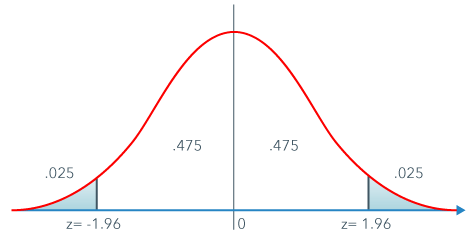Figure 10.7: Proportion of area in the critical region of the standard normal curve for α= 0.05​​​

In the example above, the critical value of z is the z-score that cuts off 2.5% of the area under the standard normal, or z-score, distribution at each tail of the distribution. As we have seen previously, z-scores of -1.96 and +1.96 are the cut-off points for a two-tailed test with an alpha or probability level of .05.

Step 5: Calculate the test statistic.

Begin with writing the formula for the test. The standard z-score formula for hypothesis testing of single samples is written as follows:

where x̅ is the sample mean, μ is the population mean, and σ is the standard error of the mean.

Let’s write down what we know:

The sample mean x̅ = 255 minutes per day,

x̅ = 255

The population mean μ = 278.67 minutes per day,

μ = 278.67

The standard error of the mean σ = 9.79,

σ = 9.79

Enter the known values into the z-score formula for single–sample testing.

Tip for working with mathematical formulas: Write the symbol for the statistic you are computing on the left and then the computational formula for the statistic. Keep equal signs under equal signs to organize your work.

Step 6: Compare the calculated statistic to the critical value and decide whether to reject or fail to reject the null hypothesis.

We have finished the math computations and obtained our single-sample z-score. Let’s take a moment to look at the value and sign of the z-score and see if the obtained score makes reasonable sense. The average number of minutes spent using a cell phone in the population was 278.67. The average daily cell phone usage in our hypothetical university sample was 255 minutes. Since the mean number of minutes in our sample is less than the population mean, we would expect a negative z-score, because subtracting a larger number from a smaller number results in a negative answer. In other words, the z-score, or one-number-statistic, which we will use to evaluate our hypothesis, confirms that our sample mean was below the expected population mean. We now need to determine if the sample mean is so far below the population mean that we would expect to draw a sample with a mean that low from the population less than 5% of the time by chance. This last sentence is very important for understanding hypothesis testing, so read it again carefully to make sure you understand the logic behind it.

In this example a z-score of -2.42 is definitely beyond the cut-off z-score of -1.96. Our test statistic (the one-sample z statistic) falls in the reject H0 region. Decision: reject Hp< .05.

Step 7: Interpret the decision in terms of the original claim.

This step is critical and often missed by students. Remember that statistics are tools that researchers use to help them draw conclusions about data, so don’t forget to draw conclusions. Refer to the original data set and the null and alternative hypotheses.

We rejected H0 so the alternative hypothesis is supported, providing support to the claim that a difference in cell phone usage exists between the students at your university and the students in Lepp, Barkley, and Karpinski’s (2014) study. The students at your university spend significantly fewer minutes per day on their cell phones than did students in Lepp, Barkley, and Karpinski’s (2014) reference population.

In your interpretation of the results, be sure to specify how the sample was different!

It may not be enough to merely say that the single-sample z-score revealed that the university sample was different from the population mean. Although “different” supports the claim, “different” does not help researchers draw conclusions. Just imagine that your friend wants to set you up on a blind date with someone who is described as “different.” Hmmm, don’t you want to know how the person is different?

Use as much information as is available to you to explain how the results differ from the outcome stated in the null hypothesis.

Note: The summary statement included an explanation of “who scored higher or lower than whom on what” – this is a good general guideline for explaining the outcome of statistical tests.

P-Value Approach

Let’s return to the table of areas under the standard normal curve and a picture of the curve to compute the probability of a z-score of -2.42. If we look up a z-score of 2.42 in the z-score table we see that the area between the mean and a z-score of 2.42 = .4922, which means that the area above z = .0078 (since the sum of both numbers must = .5, half of the distribution).Figure 10.8:​ Translation of area under the standard normal curve into probability values​

Since the distribution is symmetrical, the probability of obtaining a z-score above 2.42 is the same as the probability of obtaining a z-score below -2.42. The probability, then, of obtaining a z-score of -2.42 is .0078, and the probability of obtaining a z-score above 2.42 is also .0078.

Since this was a two-tailed test, we combine those probabilities to get the P-value associated with the outcome.

The two-tailed probability of the z-score = .0078 + .0078 = .0156.

P = .02

To summarize this scenario, the probability of obtaining a mean cell phone score of 255 minutes, corresponding to a z-score of -2.42 (or a score 2.42 standard deviations below the population mean) is .0156. Since this P-value is below the limit of .05 set by alpha, the null hypothesis can be rejected; there is less than a .05 probability the results were due to chance. According to APA style standards, the result would be rounded to two significant digits and reported as “P = .02.”

Good statistical practice is to report the P-value of the outcome either in the text of the research report or with the summary of the results. This is in line with the need to use as much information as is available to you in summarizing and reporting results.

Critical Values of z

Critical values are those values of a standardized test statistic that cut off rejection (critical) regions of the distribution being used for the statistical test. For reference, commonly used critical values for z are given in the table below.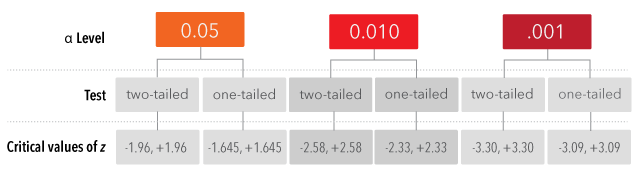Figure 10.9: Commonly used critical values of the standard normal curve​​

For each reported single-sample z-statistic below, state whether the correct decision would be to reject H0 or fail to reject H0.

10.06

One-tailed test with α = .01, z = -0.67

A

Reject

B

Fail to reject

10.07

Two-tailed test with α = .05, z = 2.36

A

Reject

B

Fail to reject

10.08

One-tailed test with α = .05, P-value of outcome = .035

A

Reject

B

Fail to reject

10.09

Two-tailed test with α = .01, P-value of outcome = .035

A

Reject

B

Fail to reject

Small Sample Testing Using the t-Test

In the real world of hypothesis testing, most often information about variability is lacking, e.g., the standard deviation of the population is usually not known and sample sizes may be too small to be able to estimate it accurately. Why can small sample sizes be misleading? Watch the following video to see an example:

You can still make a comparison between the sample mean and population mean for smaller samples, but you will need to use a different statistical tool: the one sample t-test, based on the t-distribution.

Recall that the t-distribution is actually an entire family of distributions, unlike the standard normal distribution, which is a single distribution. Each t-distribution is determined by the degrees of freedom associated with it, which in turn is determined by the sample size n.Figure 10.10: ​Effect of sample size on the shape of the standard normal curve

Calculating a t-Score

The formula for t is essentially the same as for z, except that s is used as a best estimate of the unknown σ.

Critical Values of t-Distributions

As you learned previously, the t-distributions are quite similar to the standard normal curve, or z-score distribution. Whereas the z-score curve is fixed, with a standard shape, area, and cut-off scores (e.g. ±1.96 cuts off 2.5% of the area on each end of the curve), the t-distributions are a family of distributions. Each t-distribution has a normal curve-like appearance except that the shape of the curve changes with the sample size. Thus the critical values that cut off areas of the curve corresponding to particular values of alpha are not constant, as they are with z-scores, but vary as a function of the degrees of freedom associated with a particular scenario.

Since the critical values depend on the df they must be either calculated or looked up in a table. It is usually best to simply look up the critical t-score in a table.

Compute the degrees of freedom and look up the critical values of t for each problem below. Round the critical value to two decimal places.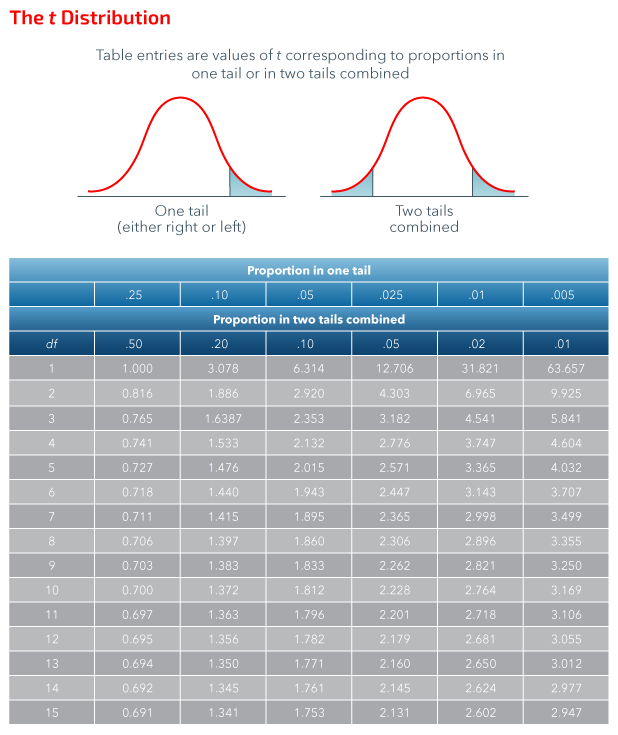Figure 10.11: Proportions of Areas in t-Distributions

10.10

α= .05; 2-tailed, n=14

Premise
Response
1

$df$

A

13

2

t$_{crit}$

B

2.145

C

28

D

14

E

2.160

F

2.131

10.11

α=.01; 2-tailed; n=8

Premise
Response
1

$df$

A

8

2

t$_{crit}$

B

4

C

3.499

D

3.355

E

4.604

F

7

10.12

α=.05; 1-tailed; n=12

Premise
Response
1

$df$

A

12

2

t$_{crit}$

B

11

C

10

D

1.796

E

1.812

F

1.782

10.13

Match the alpha level and degrees of freedom with the appropriate critical t-value; all tests are two-tailed.

Premise
Response
1

α and ($df$)

A

.05 (5)

2

Critical $t$

B

3.747

C

.05 (6)

D

.05 (4)

E

1.943

F

2.571

10.14

Match the alpha level and degrees of freedom with the appropriate critical t-value; all tests are two-tailed.

Premise
Response
1

α and ($df$)

A

2.262

2

Critical $t$

B

.05 (10)

C

2.718

D

0.5 (11)

E

.05 (9)

F

1.812

10.15

Match the alpha level and degrees of freedom with the appropriate critical t-value; all tests are two-tailed.

Premise
Response
1

α and ($df$)

A

0.05 (15)

2

Critical $t$

B

2.131

C

0.05 (14)

D

1.761

E

0.05 (13)

F

2.650

Did you notice a relationship between the degrees of freedom (df) and the critical t-value? The larger the df the smaller the critical t!

Example

A group of local fishermen is having a discussion about the size of the trout they’ve been catching in nearby Lake Mechamu. Fisher Tom thinks his fish have been smaller than usual, while Alice thinks her fish have been longer than usual. They decide to start recording the length of trout they catch and test whether or not, based on their sample, the trout in the lake differ significantly in size from previous years. The Department of Natural Resources reported that, based on last year's catch records, the average length of trout in the lake was 15.6 inches; the report did not include the standard deviation of the measurement. Members of the group fish for the next several weeks, procure a sample of 15 trout, and record the length of each:

10, 8, 6, 10, 15, 12, 14, 15, 18, 12, 17, 16, 9, 17, 18

Sam, a member of the fishermen’s group who is taking Introduction to Statistics at his local community college, volunteers to carry out the data analysis, and works through the steps below.

Step 1: State the claim and identify the null (H0) and alternative (H1) hypotheses.

The null hypothesis states that the average size of the fish that Sam’s friends caught is equal to the population parameter of 15.6 inches. The alternative hypothesis states that the average size of the fish that they caught is not equal to 15.6 inches. This is a two-tailed test; i.e., a significant result in either direction would provide support for the alternative hypothesis.

Step 2: Determine the alpha level (α).

Sam decides to test the hypothesis at a probability level of .05, so α = .05.

Step 3: Determine the appropriate test to use.

Since Sam knows the population mean (μ), but does not know the population standard deviation (σ), and since the sample size is small (n =15), he determines that the appropriate test is the single-sample t-test.

Step 4: Identify the critical value of the test statistic to indicate under what condition the null hypothesis should be rejected or not rejected.

The information needed to look up the critical value of t is

• the α-level or probability level, which was set at Step 2 as α = .05,
• the degrees of freedom, computed here as df = n – 1 = 14, and
• whether the hypothesis is one-tailed or two-tailed; the test is two-tailed.

Now you can look up the critical value of t. Below is an excerpt from a standard t-table.Figure 10.12: ​Excerpt from a standard t-test table of critical values.​

The critical value of t for a two-tailed test with α = .05 and df = 14 is ± 2.14. The logic of hypothesis testing for a single-sample t-test is identical to that for a single-sample z-test. If the single-sample t–statistic we obtain is greater than the critical value of 2.14 or less than -2.14, then our decision must be to reject H0. If our single-sample t falls between the critical values, i.e. -2.14 < t < 2.14, then we must fail to reject H0.

Step 5: Calculate the test statistic.

where x̅ is the sample mean, μ is the population mean, and σ is the standard error of the mean.

Since Sam collected raw data, we have a little work to do to find the necessary elements for the single-sample t-test. We know that μ = 15.6. We also need to find the mean, x̅, and the standard deviation, s. To find s let’s use the computational formula for the variance from Descriptive Statistics, shown here, then take the square root of the variance to find s, the standard deviation.

Start with a table of data entries: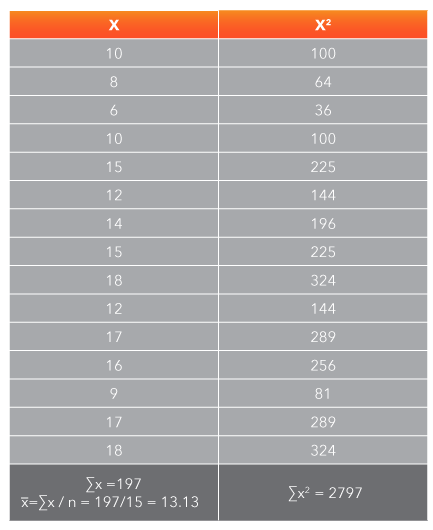Figure 10.13: Sample raw data of length of fish caught in lake Mechamu​​​​

Compute s2 and s using the computational formula from above.

Now we can compute t using the values we’ve calculated above.

Step 6: Compare the calculated statistic to the critical value and decide to reject or fail to reject the null hypothesis.

Since the obtained t-value of -2.47 exceeds the critical value of ±2.14, the statistical decision is to reject H0, p< .05.

Step 7: Interpret the decision in terms of the original claim.

Since the single-sample t-test we computed to compare the average length of fish caught by the fishers to the population mean yielded a t-score of -2.47 we reject H0. There is support for the alternative hypothesis that the lengths are different. The average length of the fish that the fishermen caught in Lake Mechamu is significantly less than the population mean of 15.56 inches (M = 13.3, SD = 3.87), t(14) = -2.47, p = .03.

P-Value Approach

Using the P-value approach with a t-test brings up a difference between the Standard Normal Table and the t-table. The Standard Normal Table gives probabilities associated with z-scores. The t-table does not give probabilities but instead gives critical values of t. Since the critical values used with t are different for each combination of df and alpha, it would require a huge number of tables to include all the possibilities. Thus the tables are condensed to include only the critical values.

The best way to obtain P-values to go with t-scores is to use technology to calculate them. As a convenience, a number of websites have online calculators available for this purpose, so you don’t necessarily need an expensive graphing calculator to figure the probabilities. There is one such (free) online calculator at the Social Science Statistics website, linked here.

Entering 14 df and the t-score of -2.47, the calculator returns a two-tailed probability value of .0270. FYI, this result works out to be the same as if you were to take the one-tailed probability of .0135 and double it to get the probability for a two-tailed outcome. Are you getting the logic? According to APA style standards, the result of .0270 would be rounded to two significant digits and reported as “p = .03.”

In this scenario, the probability of obtaining a sample with a mean length of 13.3 inches, corresponding to a t-score of -2.47, is .03. Since this P-value is below the limit of .05 set by alpha, the null hypothesis can be rejected.

Recall that good statistical practice is to report the P-value of the outcome either in the text of the research report or with the summary of the results. This is in line with the need to use as much information as is available to you in summarizing and reporting results.

For the scenarios below, indicate whether you would use a z-test or a t-test, and whether the test would be two-tailed, left-tailed, or right-tailed.

10.16

A researcher is interested in whether the reaction time (RT) to a signal change of a sample of 24 student drivers is slower (greater RT) than the RT published in a news report.

A

$t$-test, two-tailed

B

$t$-test, right-tailed

C

$t$-test, left-tailed

D

$z$-test, two-tailed

E

$z$-test, right-tailed

F

$z$-test, left-tailed

10.17

A clinical psychologist wants to compare the scores of his therapy group of 18 clients on a measure of self-confidence to the results in a published paper that includes the mean and standard deviation.

A

$t$-test, two-tailed

B

$t$-test, right-tailed

C

$t$-test, left-tailed

D

$z$-test, two-tailed

E

$z$-test, right-tailed

F

$z$-test, left-tailed

10.18

A social worker intends to survey a sample of 20 households in a neighborhood in a predominantly low-income area in order to see if the average household income is above or below the poverty level figures published by the federal government.

A

$t$-test, two-tailed

B

$t$-test, right-tailed

C

$t$-test, left-tailed

D

$z$-test, two-tailed

E

$z$-test, right-tailed

F

$z$-test, left-tailed

Testing a Hypothesis About a Proportion

Sometimes our data set involves proportions, rather than scores on a dependent variable.

In this case a z-test can be used to test a hypothesis about a population proportion P for a binomial distribution. A binomial, as you have seen, has two possible outcomes, termed either a success or failure, with P representing the probability of a success and (1 - P) representing the probability of a failure on any given trial.

To use z, the population must, as always, be approximately normally distributed. In the case of a binomial, the population can be assumed to be normally distributed as long as the product of the number of trials n and the probability of a success and of a failure on a given trial are both equal to five or more. If the distribution meets that test, then the sampling distribution for the sample proportion (pronounced p-hat) is approximately normal.

Since we are testing a hypothesis about a proportion, the test statistic is , the sample proportion and the standardized test statistic is z.Figure 10.14:​​ Requirements and formula for the z-test for proportions​​

Example: Is There Bias in Favor of the Number Seven?

For a class project in her statistics class, a student wants to investigate whether students at the college tend to favor the number seven when picking numbers from a group. In a sample of 371 students, 45 chose the number seven when picking a number between one and twenty “at random.” Does this provide convincing statistical evidence of bias in favor of the number seven, in that the proportion of students picking seven is significantly higher than 1/20 = .05? Use a significance level of .01.

Step 1: State the claim and identify the null and alternative hypotheses.

• The claim is that there is bias in favor of selecting the number seven.
• H0: p ≤ .05
• H1: p > .05

Step 2: Specify the level of significance.

• α = .01

Step 3: Determine the appropriate test to use.

• p = .05, the probability of selecting number 7
• q = 1 – p = .95, the probability of not selecting 7
• Both np and nq ≥ 5, so the sampling distribution for is approximately normal.
• Use the z-test for proportions.

Step 4: Identify the critical value of the test statistic.

• The critical value for a right-tailed test is z = 2.33

Step 5: Calculate the test statistic.

• Find , the proportion of selections that are equal to the number 7.

= x / n

p̂ = 45 / 371

p̂ = .12.

• Next, to calculate z, write down the formula and substitute the values of , p, q, and n.

Step 6: Compare the calculated value to the critical value and decide whether to reject the null hypothesis.

The calculated value of z exceeds the critical value of 2.33, so reject the null hypothesis.

Step 7: Interpret the decision in terms of the original claim.

There is evidence of bias in favor of the number seven. Specifically, the number 7 is chosen more often than expected by chance (= .12, p = .05, z = 6.19, p = .0001)

Example: A Gender Gap in Psychology Degrees

According to an article published by the American Psychological Association in gradPSYCH Magazine, women receiving doctoral degrees in psychology outnumber men 3 to 1. In one social sciences statistics course, of the 25 psychology majors there are 18 women and 7 men. We want to know if the gender ratio of psychology majors in this course is different from the population gender ratio reported by the author. Both the sample data and the population data are proportions, not means.

We can test whether a sample proportion differs from a population proportion using a single-sample z-test. The logic of the single-sample z-test for proportions is identical to the single-sample z-test for means, but the formula looks a bit different. The numerator of the z-statistic formula is the difference between the sample proportion and the population proportion. The denominator of this z-statistic estimates the standard error of the difference between the sample proportions under the assumption that the two population proportions are equal, i.e., under the assumption that H0 is true.

Step 1: State the claim and identify the null and alternative hypotheses.

According to the APA, the population proportion of women to men earning psychology doctorates is 3 to 1. In other words, for every 4 people who receive a doctorate in psychology, 3 of the 4 are women. Written as a percentage, 75% are women; written as a proportion, .75 are women. So the population proportion, symbolized by p, is equal to .75. The null hypothesis states the proportion in this class is equal to the population proportion, while the alternative states the proportion is not equal to the population proportion.

• H0: p = .75
• H1: p ≠ .75

Step 2: Specify the level of significance.

• α = .05

Step 3: Determine the appropriate test to use.

• p = .75, the population proportion of women
• q = 1 – p = .25, the population proportion of men
• Both np and nq ≥ 5, so the sampling distribution for is approximately normal.
• Use the z-test for proportions.

Step 4: Identify the critical value of the test statistic.

• The critical value for a two-tailed test is z = ±1.96

Step 5: Calculate the test statistic.

• Find , the proportion of women in the class.

= x / n

= 18 / 25

= 0=.72

• Next, to calculate z, write down the formula and substitute the values of , p, q and n.

Step 6: Compare the calculated value to the critical value and decide whether to reject the null hypothesis.

The calculated value of z does not exceed the critical value of -1.96, so fail to reject the null hypothesis.

Step 7: Interpret the decision in terms of the original claim.

There is no evidence that the gender ratio in the class is different from the published population proportions ( = .72, p = .75, z = -0.35, p = .7264).

Hypothesis Testing Steps Summary

Congratulations! You have learned three different single-sample statistical tests:

• the single-sample z-test for comparing a sample mean to a population mean, when σ is known or the sample is large
• the single-sample t-test for small samples, when σ is not known
• the single-sample z-test for comparing a sample proportion to a population proportion.

Regardless of which test we conducted, the hypothesis testing steps were the same. Let’s review the steps.

Step 1: State the claim and identify the null (H0) and alternative (H1) hypotheses.

For comparing a single-sample mean to a population mean, H0 is most often written as

The mean of the sample is equal to the mean of the population. Although the appropriate test will differ depending on whether or not the population standard error of the mean (σ) is known, H0 will not differ.

For testing the proportion of a single-sample against a population proportion, H0 is written as:

Step 2: Determine the alpha or probability level of your statistical test.

The probability level must be set before you conduct your statistical test. The probability level is chosen based on standard field practices and/or experimental logic.

Step 3: Determine the appropriate test to use.

For single samples, if the comparison is between means with a large sample and/or σ is known, choose the single-sample z-test. For small samples when σ is not known, conduct the single-sample t-test. For comparing proportions, conduct the single-sample z-test for proportions.

Step 4: Identify the critical values of the test statistic.

Identify the critical value(s) from the sampling distribution of your statistic (e.g. z-score or t-score distribution).

Step 5: Conduct the statistical test.

Start by locating the appropriate formula for the appropriate statistical test, determined in step 3. Note whether you are starting with raw data or summary data. If you have raw data, you may have to complete some preliminary data processing steps (such as means, standard deviations, or proportions) to find all of the terms in the statistical test formula. Work very slowly and carefully here. Keep equals under equals, and do not round intermediate computational steps.

Step 6: Compare the calculated statistic to the critical value and decide to reject or fail to reject the null hypothesis.

Regardless of the statistical test you are computing, the logic is always the same. Compute a single-sample statistic to test the null hypothesis. Identify the critical value from the sampling distribution of your statistic (e.g. z-score or t-score distribution). Compare the answer you obtain against the statistic’s sampling distribution critical value. If the computed test statistic value is beyond the critical value, reject H0. If the computed test statistic does not exceed the critical value, then fail to reject H0.

Step 7: Interpret the decision in terms of the original claim.

Summarize the finding in words. This is the final and most important step. Statistics are tools that researchers use to draw conclusions about hypotheses. A lone statistical value (e.g. t = 4.5) has no meaning out of context. Go back to the original question. What did you want to know? What was the sample size? When summarizing a reject H0 finding in words, be sure to explain “who scored higher or lower than whom on what.” Avoid ambiguous explanations like stating that the sample was “different” from the population.

Conclusion

In this chapter, you learned that single sample statistical tests are used to compare sample statistics to population parameters, most often reported in scientific literature. When comparing sample means (x̅) to the population mean (µ) with sample sizes ≥ 30, the single-sample z-test should be used; with sample sizes <30, the single-sample t-test is the appropriate test to use. Lastly, we learned how to use the z-test for proportions to compare a single-sample to a population when the data consist of proportions, rather than means. For a refresher, review the worked-through examples of each test and the case study, and watch the video demonstrations. You should have a firm understanding of the hypothesis testing steps before proceeding to Two-sample Hypothesis Testing, which will build on the information learned in this chapter.

Case Study: Autism Prevalence

The following case study will allow us to apply and interpret the z-test for proportions to a real-world problem. According to a recent article on the prevalence of autism spectrum disorders in the United States published by Live Science, 1 in 45 children in the United States has an autism spectrum disorder. The article cites data from a Centers for Disease Control and Prevention (CDC) study that included data from the years 2011 to 2014. The author states that the number of cases of autism is significantly higher than the CDC’s previous estimate of 1 in 68 children with an autism spectrum disorder. Convert the prevalence of autism spectrum diagnoses to proportions. The sample size for the 1 in 45 proportion is given as 12,000; the sample size for the 1 in 68 proportion is given as 11,000. The author states that the difference is significant, but no z-scores or probability values are included. What do you conclude? Is the increase in the prevalence of autism significant?

To set up the problem, consider the newest data (1 in 45 children; n = 12,000) as the sample and the former CDC data (1 in 68 children) as the population proportion.

To compute the z for proportions use the formula:

where= the sample proportion, p = the population proportion, and n = the sample size

Case Study Question 10.01

Case Study Question 10.01

Does the z-statistic support the author’s claim that a prevalence rate of 1/45 children with diagnoses of autism spectrum disorders is a significant increase over the CDC’s previous level of 1/68 children?

Click here to see the answer to Case Study Question 10.01.

Case Study Question 10.02

Case Study Discussion 10.02

What are some possible problems in the way that data were collected that may lead to misinterpretations of the data?

Click here to see the answer to Case Study Question 10.02.

Case Study Question 10.03

Case Study Question 10.03

Pay particular attention to the operational definition of autism. How was autism measured in each sample?

Click here to see the answer to Case Study Question 10.03.

Pre-Class Discussion Questions

Class Discussion 10.01

Class Discussion 10.01

Why is it necessary to determine the alpha level before conducting the statistical test?

Click here to see the answer to Class Discussion 10.01.

Class Discussion 10.02

Class Discussion 10.02

If a researcher set out to test a left-tailed hypothesis, but the outcome of the statistical test is such that a right-tailed hypothesis would have been significant, can the researcher make the decision to reject H$_0$? Why or why not?

Click here to see the answer to Class Discussion 10.02.

Class Discussion 10.03

Class Discussion 10.03

Why do the critical values of t, but not z change with the sample size?

Click here to see the answer to Class Discussion 10.03.

Class Discussion 10.04

Class Discussion 10.04

How do I choose when to use a single-sample t-test instead of a single-sample z-test?

Click here to see the answer to Class Discussion 10.04.

Class Discussion 10.05

Class Discussion 11.05

Interpret the results of the following statistical analysis.

The mean preference rating for chocolate ice cream was 10.2; the mean preference rating for vanilla ice cream was 8.4. The higher the rating, the more preferred the flavor. A t-test revealed t(32) = 2.67, p=0.012. Which flavor of ice cream should you buy for a community event? Why?

Click here to see the answer to Class Discussion 10.05.This Content is Locked
Only a limited preview of this text is available. You'll need to sign up to Top Hat, and be a verified professor to have full access to view and teach with the content.

Answers to Case Study Questions

Answer to Case Study Question 10.01

The computed z-score of 6.827 clearly exceeds the critical z-value for a 1-tailed test at the 0.01 level (2.33), and supports the claim that the prevalence rate of children with diagnoses of autism spectrum disorders has increased significantly.

Answer to Case Study Question 10.02

The 1/68 proportion data were reported in 2014, but were based on data collected during 2010 from children’s medical records. The more recent data came from parent interviews. Different questions were asked at the two time points. The definition of autism was different at the two time points. The stigma for autism decreased over time, which may have lead to an increase in diagnosed and reported cases.

Answer to Case Study Question 10.03

In the past, autism was diagnosed as a variety of conditions including developmental delay, intellectual disability, and mental retardation. In the early studies, the parents were asked open-ended questions. So the actual incidence of autism may have been significantly higher, but confusion in the terminology artificially deflated the rates of autism spectrum disorder diagnoses, as defined in 2014. In the later studies, autism was a choice in a checklist of disorders, from which parents could choose.

Answers to Pre-Class Discussion Questions

Answer to Class Discussion 10.01

The alpha level should be set based on theoretical reasons and the seriousness of making a Type I error. Choosing the alpha level after the statistic has been computed most likely would lead to letting the data, instead of the theory, drive the alpha-level decision.

Answer to Class Discussion 10.02

No. Hypotheses are developed from theory, not from data.

Answer to Class Discussion 10.03

The z-distribution, or the standard normal curve is single theoretical, standard normal distribution. The t-distribution is actually a family of distributions. The shape of the t-distribution flattens out when sample sizes are small. As a result the standard score that cuts-off the critical region for statistical tests, changes with the sample size. The t-distribution is a more valid test to use with small sample sizes.

Answer to Class Discussion 10.04

The single-sample z-test requires a sample size larger than 30 and the population standard deviation must be known. The single-sample t-test should be used when sample size is < 30 OR when the population standard deviation is not known.